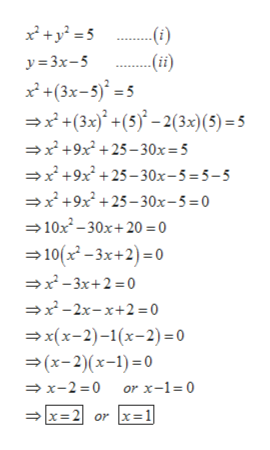# Give the solution to the system of equations. x2+y2=5, y=3x-5

Question
1 views

Give the solution to the system of equations.

x2+y2=5, y=3x-5

check_circle

Step 1

Give the solution to the system of equations.

x2+y2=5, y=3x-5

Step 2

Given

Step 3

Substituting (ii) in (i) ...help_outlineImage Transcriptionclosex+y? = 5 -(i) -(ii) y = 3x-5 x* +(3x-5)° = 5 =x* +(3x)° +(5)° – 2(3x)(5) = 5 →x +9x² +25 –- 30x=5 =x +9x² +25– 30x-5=5-5 =x +9x +25– 30x-5=0 →10x - 30x+20 = 0 = 10(x - 3x+2) =0 =x -3x+2 = 0 =x -2x-x+2 =0 = x(x-2)-1(x-2) =0 =(x-2)(x-1) =0 = x-2 =0 or x-1=0 x=2 or r x=1 fullscreen

### Want to see the full answer?

See Solution

#### Want to see this answer and more?

Solutions are written by subject experts who are available 24/7. Questions are typically answered within 1 hour.*

See Solution
*Response times may vary by subject and question.
Tagged in

### Other